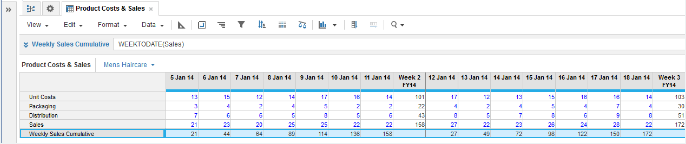1. Calculation functions
2. All Functions
3. Time and Date Functions
4. WEEKTODATEReturns cumulative sums across a week time range from a single numeric parameter as source then resets.

## Syntax

`WEEKTODATE(x)`

where:

• x: Numeric line item or a numeric value that is an expression result, such as:
`WEEKTODATE(x +1)`
`WEEKTODATE(MAX(a,b) + 27 * 6)`

## Format

Input Format Output Format

x: Line item

Number

## Arguments

The function uses the following arguments:

• x: Number: Numeric line item or property

## Constraints

The function has the following constraints:

• Time period granularity of source must be Day.
• Cannot be used if Calendar Type is Weeks: General or Calendar Months/Quarters/Years.

## Excel equivalent

• No Excel equivalent

## Example

WEEKTODATE is used to show cumulative sales figures for each week period for the first two full-week periods of a Weeks: 4-4-5 timescale in 2014:

`WEEKTODATE(Sales)        `